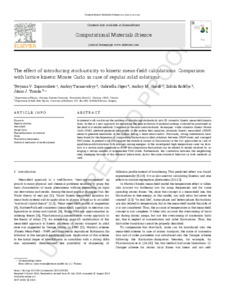# The effect of introducing stochasticity to kinetic mean-field calculations: Comparison with lattice kinetic Monte Carlo in case of regular solid solution

Zaporozhets, T. V. and Taranovskyy, A. and Jáger, G. and Gusak, A. M. (2019) The effect of introducing stochasticity to kinetic mean-field calculations: Comparison with lattice kinetic Monte Carlo in case of regular solid solution. Computational Materials Science. pp. 1-7.Preview
Text
commat_proof (1).pdfView Item